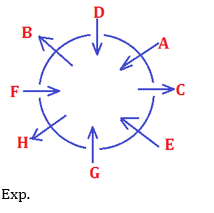# RRB PO Prelims Reasoning Ability Quiz – 10

## RRB PO Prelims Reasoning Ability Quiz

Almost all major competitive exams in the country have a section on Reasoning Ability, and it is also one of the most scoring sections. Candidates can ace this section in exams if they practice regularly in a dedicated manner. So here we are providing you with the RRB PO Reasoning Ability Quiz to help you prepare better. This RRB PO Reasoning Ability Quiz includes all of the most recent pattern-based questions, as well as Previous Year Questions. This RRB PO Reasoning Ability Quiz is totally FREE. Candidates will be provided with a detailed explanation of each question in this RRB PO Reasoning Ability Quiz. Candidates must practice this RRB PO Reasoning Ability Quiz to achieve a good score in the Reasoning Ability Section.

Directions (1-5): Read the information given below and then answer the questions that follow.
Eight friends A, B, C, D, E, F, G and H are sitting around a circle in such a way that three of them are facing outside and rest of them are facing the centre.
E is 2nd to the left of H, who is facing outside. D is second to the left of F and third to the right of E, who is facing the centre. B is third to the left of G and faces outside. G is third to the left of A, who is not an immediate neighbor of E and F. The friends facing outside are not adjacent.

1. Which of the following group is facing outside?
(a) BHE
(b) CBH
(c) CDH
(d) cannot be determined
(e) None of these

Ans. b2. What is D’s position with respect to A?
(a) Immediate right
(b) 3rd to the left
(c) Opposite
(d) 2nd to the right
(e) Immediate left

Ans. a3. Who is 2nd to the right of B?
(a) H
(b) G
(c) C
(d) A
(e) D

Ans. d4. Who is sitting third to the right of C?
(a) E
(b) F
(c) C
(d) A
(e) H

Ans. e5. Which of the following is true about the given information?
(a) C and F are facing each other
(b) E is third to the left of F
(c) C is third to the left of B
(d) Two persons are sitting between G and A, if counted in clock wise direction from A.
(e) None of these

Ans. dDirections (6-10): Study the following information to answer the given questions.Ans. d
Exp. I.A<B (False) II.E≥B (False)Ans. a
Exp. I. Q > S (True) II. Q = T (False)

8. Statements:
G≥F>A=C<D, A=B≥E<H
Conclusions:
I.A≤G
II.D>E
(a) Only I is true
(b) Only II is true
(c) If either I or II follow
(d) If neither I or II follow
(e)If both I and II follow

Ans. b
Exp. I.A≤G (False) II.D>E (True)

9. What should be placed in place of question mark in equation B ≤ A ? N ? K ? S to make A > S always true.
(a) =, >, ≥
(b) =, <, ≥
(c) =, >,<
(d) =, >, ≤
(e) None of these

Ans. a
Exp. =, >, ≥

10. Statements:
P > Q ≥ R, T = R ≤ A, A ≥ B
Conclusions:
I. B < P
II. P ≤ B
(a) Only I follows
(b) Only II follows
(c) Either 1st or 2nd
(d) Neither 1st nor 2nd
(e) Both 1st and 2nd

Ans. c
Exp. I. B < P (False) II. P ≤ B (False)###Buy Bank MahaCombo Package

### Preparation Kit PDF

#### Most important PDF’s for Bank, SSC, Railway and Other Government Exam : Download PDF Now

AATMA-NIRBHAR Series- Static GK/Awareness Practice Ebook PDF Get PDF here
The Banking Awareness 500 MCQs E-book| Bilingual (Hindi + English) Get PDF here
AATMA-NIRBHAR Series- Banking Awareness Practice Ebook PDF Get PDF here
Computer Awareness Capsule 2.O Get PDF here
AATMA-NIRBHAR Series Quantitative Aptitude Topic-Wise PDF 2020 Get PDF here
Memory Based Puzzle E-book | 2016-19 Exams Covered Get PDF here
Caselet Data Interpretation 200 Questions Get PDF here
Puzzle & Seating Arrangement E-Book for BANK PO MAINS (Vol-1) Get PDF here

3